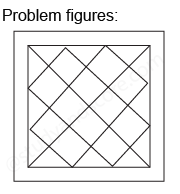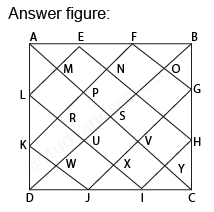## Practice Test Discussion

Q)

In each of the following question, find the minimum number of triangles in the given figure.• ExplanationAfter labelling the figure, we get the following,

• Simplest triangles AML, LRK, KWD, DWJ, JXI, IYC, CYH, HTG, GOB, BOF, FNE, and EMA (12)
• Triangles with two components each AEL, KDJ, HIC, and FBG (4)
• Triangles with three components each APF, EQB, BQH, GVC, CVJ, IUD, DUL, and KPA (8)
• Triangles with six components each ASB, BSC, CSD, DSA, AKF, EBH, GCJ and IDL (8)
• Triangles with twelve components each ADB, ABC, BCD and CDA (4)

Total number of triangles in the figure is 12 + 4 + 8 + 8 + 4 = 36

Hence option C is correct.

- Share with your friends! -